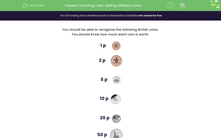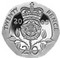# Count Coins of Different Values

In this worksheet, students will count the total value of a range of coins under 1 pound in value.Key stage:  KS 1

Curriculum topic:   Measurement

Popular topics:   Money worksheets

Difficulty level:#### Worksheet Overview

You should be able to recognise the following British coins - otherwise, shopping could be a bit of a problem!You should know how much each coin is worth.

1p2p5p10p20p50pExample

Add up the total value of these coins in pence.In pence, we have 1 + 1 + 2 + 2 + 2 + 5 + 10 + 20 + 50.

50 + 20 = 70

70 + 10 = 80

80 + 5 = 85

85 + 2 + 2 + 2 = 85 + 6 = 91

91 + 1 + 1 = 91 + 2 = 93p

Sometimes, it is easier to add up the totals of each type of coin, starting with the largest.

so we have... 50 + 20 + 10 + 5 + 6 + 2 = 93p

Are you ready to have a go?

### What is EdPlace?

We're your National Curriculum aligned online education content provider helping each child succeed in English, maths and science from year 1 to GCSE. With an EdPlace account you’ll be able to track and measure progress, helping each child achieve their best. We build confidence and attainment by personalising each child’s learning at a level that suits them.

Get started